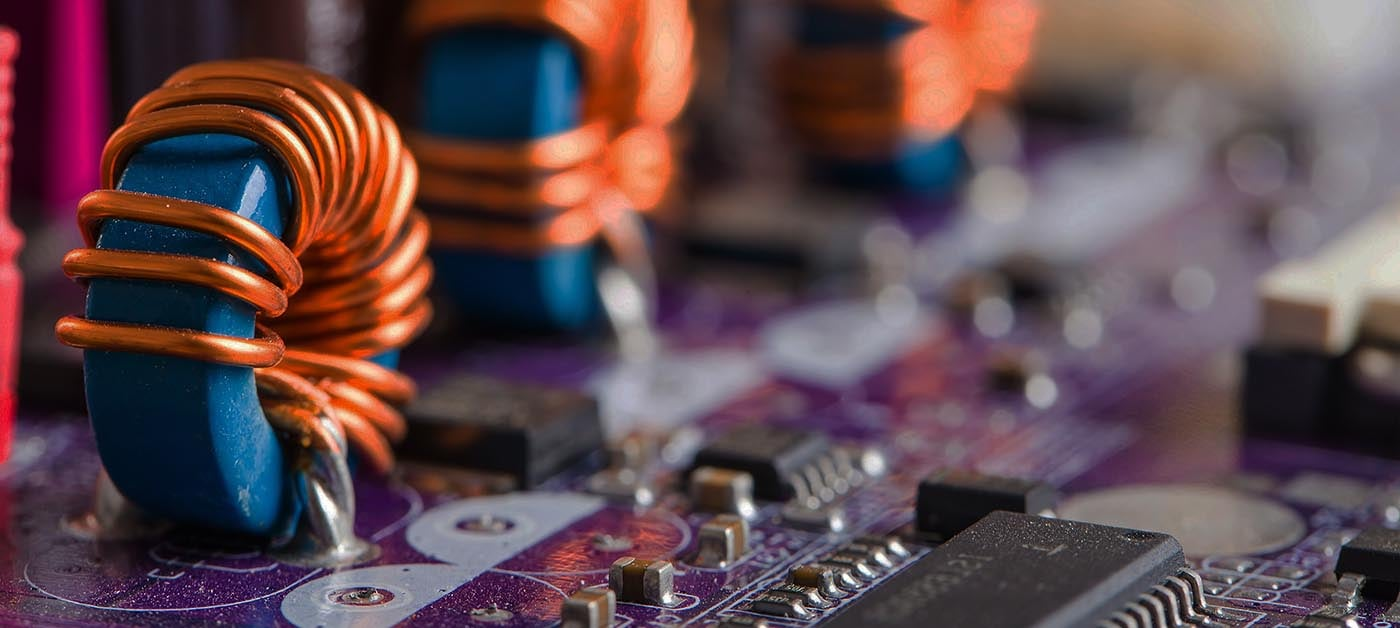# MATLAB-Simulink Course: Power Electronics Simulations

Learn how to simulate any power electronics device in MATLAB/Simulink, including rectifiers, DC-to-DC converters, and inverters (single-phase and three-phase). It consists of 4 sections, 30 lectures in 6h 22m total course length.

Current Status
Not Enrolled
Price
\$28.45
Get Started

## Course Description

This course is designed to allow you to simulate any power electronics device in MATLAB/Simulink, including rectifiers, DC-to-DC converters, and inverters. This course not only gives a review of the theory of how rectifiers, DC-to-DC converters, and inverters work but also gives several examples of how to simulate these devices using MATLAB/Simulink.

The MATLAB/Simulink models for the power electronics devices created during the lectures are available for download with each lecture.

The course is divided into the following sections:

### 1. Introduction to MATLAB/Simulink for Power Electronics

In the first section, we will begin by reviewing the theory behind the semiconductor devices that are used in power electronics, such as diodes, power BJTs, power MOSFETs, IGBTs, and Thyristors. We will then take a look at the libraries available in Simulink to represent these devices in our models. We will learn how to model voltage sources, current sources, and passive components (resistors, capacitors, and inductors), as well as how we can put them all together in a model using Simulink. We’ll also learn how to take measurements in the model to ensure proper simulation.

### 2. Rectifier Simulations in MATLAB/Simulink

The second section will start with reviewing the theory behind the operation and topologies of power electronics rectifiers. We will then see how we can simulate both single-phase and three-phase rectifiers using Simulink.

### 3. DC-to-DC Converter Simulations in MATLAB/Simulink

We will begin section 3 by reviewing the theory behind the operation and topologies of power electronics DC-to-DC converters. We will learn how to simulate buck, boost, and buck/boost converters.

### 4. Inverter Simulations in MATLAB/Simulink

The fourth section will start with reviewing the theory behind the operation and topologies of inverters. We will then see how we can simulate single-phase and three-phase inverters.

As mentioned above, in each section, we will go over several models to illustrate how we can design and simulate power electronics devices in MATLAB/Simulink. The models are also available for download so that you can follow along, as well as use these models and modify them to create your own designs.

By learning how to simulate power electronics devices in MATLAB/Simulink, you will be able to further your career in electrical engineering and power electronics.

## Course Summary

1. How to simulate power electronics devices in MATLAB/Simulink
2. Simulation of buck, boost, and buck/boost converters in MATLAB/Simulink
3. How rectifiers, dc-to-dc converters, and inverters work
4. How to design power electronics devices to meet certain design specifications
5. How to implement a PID controller in MATLAB/Simulink
6. Simulation of half-wave and full-wave rectifiers in MATLAB/Simulink
7. Simulation of single-phase and three-phase inverters in MATLAB/Simulink
8. How to determine the performance of power electronics devices
10. Power Engineering and Electrical Engineering Simulations in MATLAB/Simulink

## Who Is This Course For

• Engineering students
• Practicing engineers
• Anybody with an interest in learning about power electronics and/or MATLAB/Simulink

## Requirements

• MATLAB/Simulink software, free trial available online

1. Boost Converter Model - Section 4 (zip)
2. Buck-Boost Converter Model - Section 4 (zip)
3. Buck Converter Model - Section 4 (zip)
4. Control Systems for Converters Model - Section 4 (zip)
5. Duty Cycle Model - Section 4 (zip)
6. Inverter Pulses Single-Phase - Section 5 (zip)
7. Inverter Pulses Three-Phase - Section 5 (zip)
8. Sample Simulink Model - Section 2 (zip)
9. Simulink Converters Model - Section 4 (zip)
10. Single-Phase Full-Wave Rectifier Model - Section 3 (zip)
11. Single-Phase Half-Wave Rectifier Model - Section 3 (zip)
12. Single-Phase-Inverter-Model - Section 5 (zip)
13. Three-Phase Full-Wave Rectifier Model - Section 3 (zip)
14. Three-Phase Half-Wave Rectifier Model - Section 3 (zip)
15. Three-Phase Inverter Model - S05 (zip)
16. Universal Bridge Rectifier Model - S03 (zip)

## Course Content

Lesson Content
0% Complete 0/3 Steps
Lesson Content
0% Complete 0/8 Steps
Lesson Content
0% Complete 0/9 Steps
Lesson Content
0% Complete 0/6 Steps

##### Ricardo Romero, PE

Hi, my name is Ricardo Romero and I am an Electrical Engineer with over ten years of experience in Power Systems. I obtained my Bachelor's and Master's degrees in Electrical Engineering from The University of North Carolina at Charlotte. I am also a licensed Professional Engineer in Power Systems in the states of Arizona, Louisiana, North Carolina, Pennsylvania, South Carolina, and Virginia in the United States. Although my professional experience is mainly in Power Systems, I am also passionate about other topics in Electrical Engineering including Analog Electronics, Control Systems, Digital Design, Digital Signal Processing, Electric Machinery, and Power Electronics.

13 Courses+28 enrolled
Not Enrolled

#### Course Includes

• 6 Lessons
• 33 Topics
• 1 Quiz
• Course Certificate